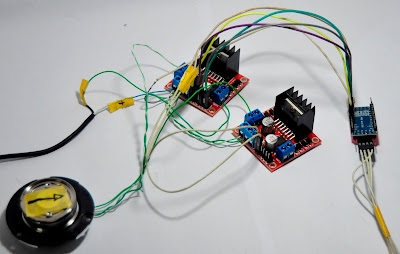## Raspberry project

This project uses L298 (known as H-bridge) to run brushless motor (taken from HDD) via Arduino Pro Mini. By this project, we also know how brushless works
Hardware needs:
1. PCB H-bridge L298 (2pcs)
2. Arduino Pro Mini
3. Brushless motor (take from HDD)1. H-Bridge L2982. Arduino Pro Mini### 1. Understand brushless motor principleImage of brushless motor of HDD
Search on web, we know that brushless motor has rotating part (called as Rotor) has no contact with electrical wire -> main feature of this motor is can run with high speed
Motor stator will make magnetic field -> when magnetic direction moving -> it will move rotor

Let's see how magnetic field moving:
Stator of motor has 3 wire, connected together at 1 point, other point of wire will connect to power source.
At "phase 1", power (+) is applied to Green coil, and power (-) is applied to Blue coil -> sum of vector magnetic field of Green coil and Blue coil will make magnetic direction as in above picture (horizontal direction) -> this magnetic field will make rotor rotating and align to magnetic direction, then stop at this direction
To move rotor, magnetic field must moving -> see "phase 2"
In "phase 2", power (+) is applied to Red coil, and power (-) is applied to Blue coil -> sum of vector magnetic field will make magnetic direction as in above picture -> this magnetic field will move rotor to this direction
Continue with phase 3, 4, 5, 6 -> magnetic field rotates 1 circle -> rotor also rotates 1 circle

### 2. Driver for brushless motor

A Driver for brushless motor is used to arrange power (+) and power (-) synchronizing to each coil Blue, Red, Green for rotating magnetic field
Following table is summarize of power (+) and power (-) need to apply each coil at each phase:
 Phase 1 2 3 4 5 6 Blue - - N + + N Red N + + N - - Green + N - - N +
Three pairs of transistor will be ON/OFF as pattern in above picture to make rotating magnetic field

### 3. Connection to L298 H-bridge

Half of H-bridge is used to connect to each coil of brushless motor

Look inside L298 IC -> it is possible to flow current from half H-bridge to another half H-bridge

### 4. Circuit connection

Schematic diagram by Fritzing:

ENA IN1 is connected to H-bridge 1
ENB IN3 is connected to H-bridge 2
ENC IN1 is connected to H-bridge 3
This project need 3 half of H-bridge  -> need 2 L298 PCB. Here is my result connection:Result of connection

For this connection, we have pattern code as here:
 Phase 1 2 3 4 5 6 Blue - - N + + N Red N + + N - - Green + N - - N + ENA 1 1 0 1 1 0 IN1 0 0 0 1 1 0 ENB 0 1 1 0 1 1 IN3 0 1 1 0 0 0 ENC 1 0 1 1 0 1 IN1 1 0 0 0 0 1
Next is coding for above table

### 5. Coding for Arduino

```/*
Motor - brushless motor control
Created 07 Jan. 2018
This example code is in the public domain.
http://engineer2you.blogspot.com
*/

const byte en_A = 2;
const byte in_A = 3;
const byte en_B = 4;
const byte in_B = 5;
const byte en_C = 7;
const byte in_C = 8;

int speed_motor = 20;

void setup() {
pinMode(en_A,OUTPUT);
pinMode(in_A,OUTPUT);
pinMode(en_B,OUTPUT);
pinMode(in_B,OUTPUT);
pinMode(en_C,OUTPUT);
pinMode(in_C,OUTPUT);

// start serial port at 9600 bps:
Serial.begin(9600);
while (!Serial) {
; // wait for serial port to connect. Needed for native USB port only
}
}

void loop() {
for (int i = 0; i<=(21-speed_motor)*5; i++) {
motor_run();
}

if (speed_motor > 2) speed_motor = speed_motor - 1; //increasing speed
Serial.println(speed_motor);  //print out speed to serial port
}

void motor_run() {
digitalWrite(en_A,1);
digitalWrite(in_A,0);
digitalWrite(en_B,0);
digitalWrite(in_B,0);
digitalWrite(en_C,1);
digitalWrite(in_C,1);
delay(speed_motor); //Phase 1
digitalWrite(en_A,1);
digitalWrite(in_A,0);
digitalWrite(en_B,1);
digitalWrite(in_B,1);
digitalWrite(en_C,0);
digitalWrite(in_C,0);
delay(speed_motor); //Phase 2
digitalWrite(en_A,0);
digitalWrite(in_A,0);
digitalWrite(en_B,1);
digitalWrite(in_B,1);
digitalWrite(en_C,1);
digitalWrite(in_C,0);
delay(speed_motor); //Phase 3
digitalWrite(en_A,1);
digitalWrite(in_A,1);
digitalWrite(en_B,0);
digitalWrite(in_B,0);
digitalWrite(en_C,1);
digitalWrite(in_C,0);
delay(speed_motor); //Phase 4
digitalWrite(en_A,1);
digitalWrite(in_A,1);
digitalWrite(en_B,1);
digitalWrite(in_B,0);
digitalWrite(en_C,0);
digitalWrite(in_C,0);
delay(speed_motor); //Phase 5
digitalWrite(en_A,0);
digitalWrite(in_A,0);
digitalWrite(en_B,1);
digitalWrite(in_B,0);
digitalWrite(en_C,1);
digitalWrite(in_C,1);
delay(speed_motor); //Phase 6
}
```
You might also like:

1.Can you post a full circuit diagram please?
What sort of speed are you getting the motor to rotate at?

1.Ok, please wait few days. I will finish it.

2.Is the schematic complete?
I needed him to finish my job and you're helping me a lot!

3.Hi, the schematic is completed. Please see section "4. Circuit connection"

2.Hello, l298 already is 2 h bridges (4 half bridges) so you only need 1 l298 A,B ad C can go to Mot 1 A,B and Mot2 A. Already tested this 3 years ago. And I would go with array manipulation, its faster and you'd end up with 6 lines of code, but be careful with the ampere!

1.2.3.Is there any difference if I use a brushless dc motor with 9 coils instead of one like yours (with 12 coils)?

1.Hi, there is no difference. Let's try to do.

4.CAN WE CONTROL THE BLDC WITH SINGLE H BRIDGE PLEASE SUGGEST ME...

1.Hi, you need 2 H-bridge to make control BLDC. Only 1 H-bridge can't work out for BLDC.
5.6.